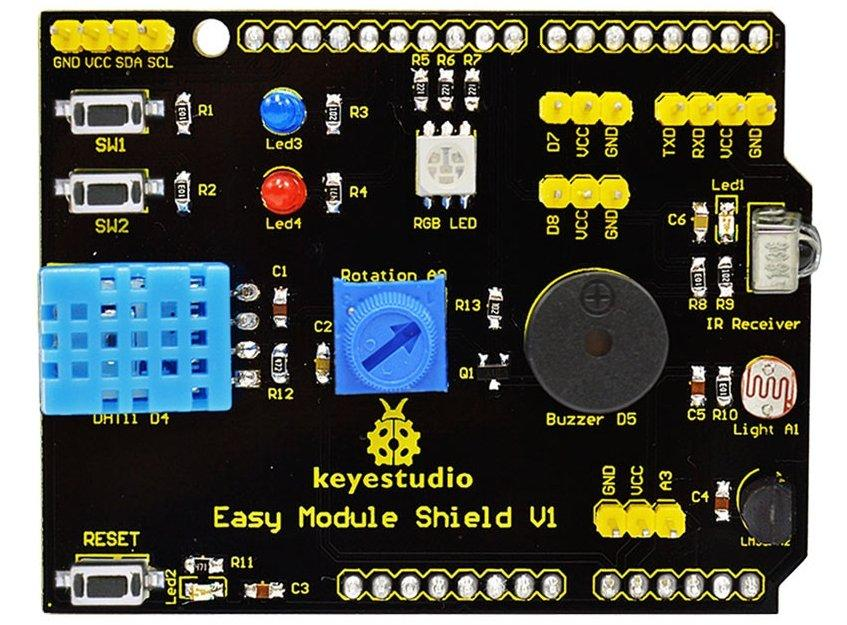# Arduino more Easy Module Shield examples

In our previous look at the Easy Module Shield v1 – Arduino Easy Module Shield v1. We took a look at the shield and had a simple example looking at the LDR and the pot. In this example we will show examples for the LM35 and the DHT11. In both examples we will output via the serial monitor but we will also switch an LED on if the temperature rises above a certain value.

Here is the shield and you can clearly see the DHT11, LM35 and the red and blue LEDs### Code

Example 1 : LM35 example

 Source code```#define lm35Pin A2
#define ledPin 12

//this sets the ground pin to LOW and the input voltage pin to high
void setup()
{
Serial.begin(9600);
pinMode(ledPin, INPUT);
digitalWrite(ledPin,0);
}

//main loop
void loop()
{
float millivolts= (rawvoltage/1024.0) * 5000;
float celsius= millivolts/10;
Serial.print(celsius);
Serial.print(" degrees Celsius, ");
Serial.print((celsius * 9)/5 + 32);
Serial.println(" degrees Fahrenheit");
if(celsius >25)
{
digitalWrite(ledPin,1);
}
else
{
digitalWrite(ledPin,0);
}
delay(1000);

}```

Open up the serial monitor and you should see something like this, put your hand on the LM35 and that should push the temperature higher than 25 degrees and the LED will light

25.39 degrees Celsius, 77.70 degrees Fahrenheit
25.88 degrees Celsius, 78.58 degrees Fahrenheit
26.37 degrees Celsius, 79.46 degrees Fahrenheit
26.86 degrees Celsius, 80.34 degrees Fahrenheit
25.88 degrees Celsius, 78.58 degrees Fahrenheit
25.39 degrees Celsius, 77.70 degrees Fahrenheit
25.39 degrees Celsius, 77.70 degrees Fahrenheit
25.39 degrees Celsius, 77.70 degrees Fahrenheit
24.90 degrees Celsius, 76.82 degrees Fahrenheit
24.41 degrees Celsius, 75.95 degrees Fahrenheit

Example 2 : DHt11 Example

 Source code```// Depends on the following Arduino libraries:
// - DHT Sensor Library: https://github.com/adafruit/DHT-sensor-library

#include <DHT.h>
#include <DHT_U.h>

#define DHTPIN 4 // Pin which is connected to the DHT sensor.
#define DHTTYPE DHT11 // DHT 11
#define ledPin 13

DHT_Unified dht(DHTPIN, DHTTYPE);

uint32_t delayMS;

void setup()
{
Serial.begin(9600);
// Initialize device.
dht.begin();
Serial.println("DHTxx Unified Sensor Example");
}

void loop()
{
// Delay between measurements.
delay(delayMS);
// Get temperature event and print its value.
sensors_event_t event;
dht.temperature().getEvent(&event);
if (isnan(event.temperature))
{
}
else
{
Serial.print("Temperature: ");
Serial.print(event.temperature);
Serial.println(" *C");
if(event.temperature >25)
{
digitalWrite(ledPin,1);
}
else
{
digitalWrite(ledPin,0);
}
}
delay(1000);
}```

Testing, open the serial monitor and try and alter the temperature on the device (blow on it). Blue led should light